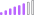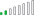cancel
Showing results for
Did you mean:Post Patron

## The function COUNT cannot work with values of type Boolean

Hi,

The code below works but it references weekday instead of the IS Working Day column which contains just a TRUE / FALSE value.

``````Total Deferral Lengthv2 =
var _mindate=
MINX(FILTER(ALL('Deferrals'),'Deferrals'[regardingobjectid]=MAX('Deferrals'[regardingobjectid])),[actualstart])
var _maxdate=
MaxX(FILTER(ALL('Deferrals'),Deferrals[regardingobjectid]=MAX('Deferrals'[regardingobjectid])),[Closed Date])
return
CALCULATE(
COUNT('Date Dimension'[Weekday]),FILTER(ALL('Date Dimension'),
'Date Dimension'[Date]>_mindate&&'Date Dimension'[Date]<=_maxdate&&NOT('Date Dimension'[Weekday] ) in {6,7}))``````

so, I have my own Date Table that contains an IS WORKING DAY (TRUE / FALSE)

When I try to amend the code above I get the COUNT cannot work with Boolean error message

``````Total Deferral Lengthv2 copy =
var _mindate=
var _maxdate=
MaxX(FILTER(ALL('Deferrals'),Deferrals[regardingobjectid]=MAX('Deferrals'[regardingobjectid])),[Closed Date])
return
CALCULATE(
COUNT('Date Dimension'[Is Working Day]),FILTER(ALL('Date Dimension'),
'Date Dimension'[Date]>_mindate&&'Date Dimension'[Date]<=_maxdate&&NOT('Date Dimension'[Is Working Day] )))``````

I would like to count the No of Working Days but I'm not sure how to?

Any help would be much appreciated.

Thanks,

1 ACCEPTED SOLUTIONPost Patron

Hi,

Just an update, your Formula works almost, I've managed to re-run it and I get no error messages now.

The only thing thats different between a calculated column that I know is 100% correct and this measure is the No of Days difference

Your formula here produced 158 days:

``````Time in Deferral =
VAR _mindate =
MINX (
FILTER (
ALL ( 'Deferrals' ),
'Deferrals'[regardingobjectid] = MAX ( 'Deferrals'[regardingobjectid] )
),
[actualstart]
)
VAR _maxdate =
MAXX (
FILTER (
ALL ( 'Deferrals' ),
Deferrals[regardingobjectid] = MAX ( 'Deferrals'[regardingobjectid] )
),
[actualend]
)
RETURN
CALCULATE (
COUNT ( 'Date'[Weekday] ),
FILTER (
ALL ( 'Date' ),
'Date'[Date] > _mindate
&& 'Date'[Date] <= _maxdate
&& 'Date'[Is Working Day] = True()))``````

But the correct number is derived from this calculated column:

``````TimeinDeferral =
VAR _Start = 'Deferrals'[actualstart]
VAR _End = 'Deferrals'[actualend]
VAR _Table = FILTER(ALL('Date'),[Date] >= _Start && [Date] <= _End && [Is Working Day] = TRUE())
RETURN
COUNTROWS(_Table)``````

7 REPLIES 7Community Support

Hi, @ArchStanton

Coluld you tell me whether your problem has been solved?

As mentioned by @OzkanDhont ,it may have something to do with the data type of your column "Is Working Day".

If the Data Type of column is 'True/false', try the formula below.``````Total Deferral Lengthv2 =
VAR _mindate =
MINX (
FILTER (
ALL ( 'Deferrals' ),
'Deferrals'[regardingobjectid] = MAX ( 'Deferrals'[regardingobjectid] )
),
[actualstart]
)
VAR _maxdate =
MAXX (
FILTER (
ALL ( 'Deferrals' ),
Deferrals[regardingobjectid] = MAX ( 'Deferrals'[regardingobjectid] )
),
[Closed Date]
)
RETURN
CALCULATE (
COUNT ( 'Date Dimension'[Weekday] ),
FILTER (
ALL ( 'Date Dimension' ),
'Date Dimension'[Date] > _mindate
&& 'Date Dimension'[Date] <= _maxdate
&& 'Date Dimension'[Weekday] =FALSE()
)
)
``````

If the Data Type of column is 'Text', try the formula below.

``````Total Deferral Lengthv2 =
VAR _mindate =
MINX (
FILTER (
ALL ( 'Deferrals' ),
'Deferrals'[regardingobjectid] = MAX ( 'Deferrals'[regardingobjectid] )
),
[actualstart]
)
VAR _maxdate =
MAXX (
FILTER (
ALL ( 'Deferrals' ),
Deferrals[regardingobjectid] = MAX ( 'Deferrals'[regardingobjectid] )
),
[Closed Date]
)
RETURN
CALCULATE (
COUNT ( 'Date Dimension'[Weekday] ),
FILTER (
ALL ( 'Date Dimension' ),
'Date Dimension'[Date] > _mindate
&& 'Date Dimension'[Date] <= _maxdate
&& 'Date Dimension'[Weekday] =FALSE()
)
)``````

Best Regards,
Community Support Team _ EasonCommunity Support

Hi, @ArchStanton

Coluld you tell me whether your problem has been solved?

As mentioned by @OzkanDhont ,it may have something to do with the data type of your column "Is Working Day".

If the Data Type of column is 'True/false', try the formula below.``````Total Deferral Lengthv2 =
VAR _mindate =
MINX (
FILTER (
ALL ( 'Deferrals' ),
'Deferrals'[regardingobjectid] = MAX ( 'Deferrals'[regardingobjectid] )
),
[actualstart]
)
VAR _maxdate =
MAXX (
FILTER (
ALL ( 'Deferrals' ),
Deferrals[regardingobjectid] = MAX ( 'Deferrals'[regardingobjectid] )
),
[Closed Date]
)
RETURN
CALCULATE (
COUNT ( 'Date Dimension'[Weekday] ),
FILTER (
ALL ( 'Date Dimension' ),
'Date Dimension'[Date] > _mindate
&& 'Date Dimension'[Date] <= _maxdate
&& 'Date Dimension'[Weekday] =FALSE()
)
)
``````

If the Data Type of column is 'Text', try the formula below.

``````Total Deferral Lengthv2 =
VAR _mindate =
MINX (
FILTER (
ALL ( 'Deferrals' ),
'Deferrals'[regardingobjectid] = MAX ( 'Deferrals'[regardingobjectid] )
),
[actualstart]
)
VAR _maxdate =
MAXX (
FILTER (
ALL ( 'Deferrals' ),
Deferrals[regardingobjectid] = MAX ( 'Deferrals'[regardingobjectid] )
),
[Closed Date]
)
RETURN
CALCULATE (
COUNT ( 'Date Dimension'[Weekday] ),
FILTER (
ALL ( 'Date Dimension' ),
'Date Dimension'[Date] > _mindate
&& 'Date Dimension'[Date] <= _maxdate
&& 'Date Dimension'[Weekday] =FALSE()
)
)``````

Best Regards,
Community Support Team _ EasonPost Patron

Hi,

Thanks for your suggestions, I've tried them all and I still get errors. (Ps I've renamed Date Dimension to Date for better clarity

For this version I get the following error message:

The function COUNT cannot work with values of type Boolean:

``````CALCULATE(
COUNT('Date Dimension'[Is Working Day]),FILTER(ALL('Date Dimension'),
'Date Dimension'[Date]>_mindate&&'Date Dimension'[Date]<=_maxdate&&'Date Dimension'[Is Working Day] = TRUE)))``````

and get this for the latter:

TRUE with () =
The syntax for ')' is incorrect. (DAX(var _mindate= MINX( FILTER( ALL ('Deferrals'), 'Deferrals'[regardingobjectid]=MAX('Deferrals'[regardingobjectid])),[actualstart])var _maxdate= MaxX( FILTER( ALL('Deferrals'), Deferrals[regardingobjectid]=MAX('Deferrals'[regardingobjectid])),[actualend])returnCALCULATE( COUNT('Date'[Is Working Day]), FILTER(ALL('Date'), 'Date'[Date]>_mindate &&'Date'[Date]<=_maxdate && ('Date'[Is Working Day] = TRUE() ) )))))Community Support

Hi, @ArchStanton

Please convert the data type of the column [Is Working Day] to 'Text' Or perform count operations on other columns whose type is not 'True/false'.then retry the formula:

``````Total Deferral Lengthv2 =
VAR _mindate =
MINX (
FILTER (
ALL ( 'Deferrals' ),
'Deferrals'[regardingobjectid] = MAX ( 'Deferrals'[regardingobjectid] )
),
[actualstart]
)
VAR _maxdate =
MAXX (
FILTER (
ALL ( 'Deferrals' ),
Deferrals[regardingobjectid] = MAX ( 'Deferrals'[regardingobjectid] )
),
[Closed Date]
)
RETURN
CALCULATE (
COUNT ( 'Date Dimension'[Weekday] ),
FILTER (
ALL ( 'Date Dimension' ),
'Date Dimension'[Date] > _mindate
&& 'Date Dimension'[Date] <= _maxdate
&& 'Date Dimension'[Weekday] = "True"
)
)
``````

Best Regards,
Community Support Team _ Eason
If this post helps, then please consider Accept it as the solution to help the other members find it more quickly.Post Patron

Hi

If I change the Binary TRUE / FALSE for IS Working Day to text then it breaks a whole load of other measures and calculations I have in my data model.

I did create a new column just to see what happens and the TRUE / FALSE turns into binary 1,s and 0's

I wrapped the "1" in quotes and a I got a result that said 15 for every record:

Total Deferral Lengthv2 = var _mindate= MINX( FILTER( ALL ('Deferrals'), 'Deferrals'[regardingobjectid]=MAX('Deferrals'[regardingobjectid])),[actualstart]) var _maxdate= MaxX( FILTER( ALL('Deferrals'), Deferrals[regardingobjectid]=MAX('Deferrals'[regardingobjectid])),[actualend]) return CALCULATE( COUNT('Date'[Is Working Dayv2]), FILTER(ALL('Date'), 'Date'[Date]>_mindate &&'Date'[Date]<=_maxdate && ('Date'[Is Working Dayv2] = "1")))Resolver II

Hi @ArchStanton !

I believe the problem resided in the usage of NOT() function.
You're trying to return all the true values in column 'Is Working Day'?

Would you mind trying following DAX?:

``````CALCULATE(
COUNT('Date Dimension'[Is Working Day]),FILTER(ALL('Date Dimension'),
'Date Dimension'[Date]>_mindate&&'Date Dimension'[Date]<=_maxdate&&'Date Dimension'[Is Working Day] = TRUE)))``````

EDIT: You might have to use TRUE() instead of TRUE.

``````CALCULATE(
COUNT('Date Dimension'[Is Working Day]),FILTER(ALL('Date Dimension'),
'Date Dimension'[Date]>_mindate&&'Date Dimension'[Date]<=_maxdate&&'Date Dimension'[Is Working Day] = TRUE())))``````

Kind regards,

ODPost Patron

Hi,

Just an update, your Formula works almost, I've managed to re-run it and I get no error messages now.

The only thing thats different between a calculated column that I know is 100% correct and this measure is the No of Days difference

Your formula here produced 158 days:

``````Time in Deferral =
VAR _mindate =
MINX (
FILTER (
ALL ( 'Deferrals' ),
'Deferrals'[regardingobjectid] = MAX ( 'Deferrals'[regardingobjectid] )
),
[actualstart]
)
VAR _maxdate =
MAXX (
FILTER (
ALL ( 'Deferrals' ),
Deferrals[regardingobjectid] = MAX ( 'Deferrals'[regardingobjectid] )
),
[actualend]
)
RETURN
CALCULATE (
COUNT ( 'Date'[Weekday] ),
FILTER (
ALL ( 'Date' ),
'Date'[Date] > _mindate
&& 'Date'[Date] <= _maxdate
&& 'Date'[Is Working Day] = True()))``````

But the correct number is derived from this calculated column:

``````TimeinDeferral =
VAR _Start = 'Deferrals'[actualstart]
VAR _End = 'Deferrals'[actualend]
VAR _Table = FILTER(ALL('Date'),[Date] >= _Start && [Date] <= _End && [Is Working Day] = TRUE())
RETURN
COUNTROWS(_Table)``````